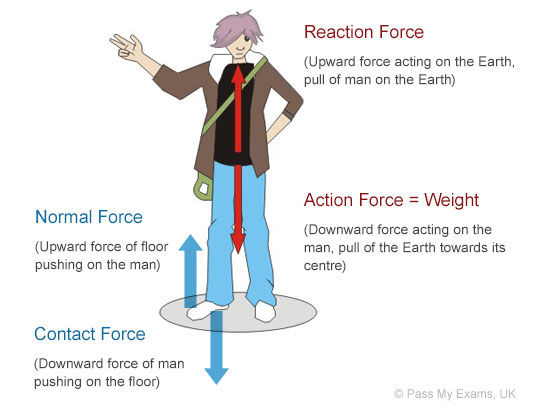## What are Forces?

A force results from the interaction between two objects. A force can be defined as a push or a pull which acts upon an object as a result of its interaction with another object.

When one object exerts a force on another object it always experiences an equal opposing force in return from the object it exerted the force on. Or in other words when two objects interact, the forces they exert on one another are equal and opposite. These forces are referred to as the action and reaction forces.

Forces are measured in units called newtons (N). The unit is named after the famous physicists Sir Isaac Newton (1642-1727) who Laws of Motion are instrumental in understanding the effects of forces.

## Examples of forces

### Weight

Weight is the force of gravity, which is the pull of the Earth on an object. To understand the force of gravity the concept of mass needs to be understood as well.

The mass of an object is the amount of matter it contains. Thus mass is a measure of how much stuff is in an object. Mass is measured in kilograms (kg) and is the same no matter where the object is located in the universe. For example and object of mass 10kg on the Earth will have the same mass of 10kg on the moon or anywhere else in the universe. Weight on the other hand is a measure of the pull of a planet i.e. Earth on the stuff contained in the object. The direction of the force of gravity is downwards towards the centre of the Earth.

As mentioned earlier when two objects interact they exert equal and opposite forces. The force that opposes the force of gravity in called the Reaction Force. This is equal to the force of gravity and acts in an upwards direction (opposite to the downwards direction of the force of gravity). This is exerted by the object on the earth.

 FREE BODY FORCE DIAGRAMS Free-body force diagrams are used to give a clear and simple indication of the effect of the forces acting on an object. In a free-body force diagram only the forces acting directly on the object are shown. The forces are represented by arrows, the direction of the arrow gives the direction of the force and the size of the arrow represents the size of the force. This assists the reader in determining the net force acting on the object.### Friction

Frictional forces are the forces that oppose or prevent motion. These forces are a result of the interaction between the surfaces of two objects (more precisely due to the attractions between the molecules of the surfaces in contact). The reason why a football that is kicked eventually comes to rest is due to the friction forces between the surface of the rolling ball and the grass and air it is in contact with.

### Magnetic Forces

This is the force produced by magnetic materials which pulls or repels other materials. For example the magnetic strip on a refrigerator door catch pulls the door to the refrigerator frame to keep the door closed.